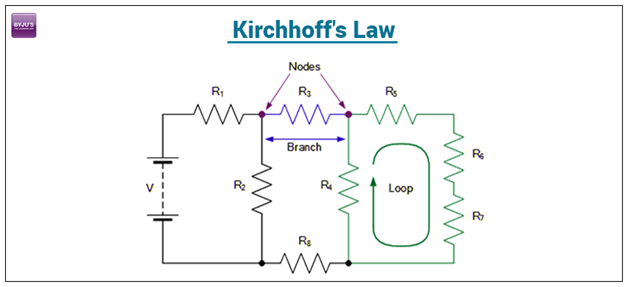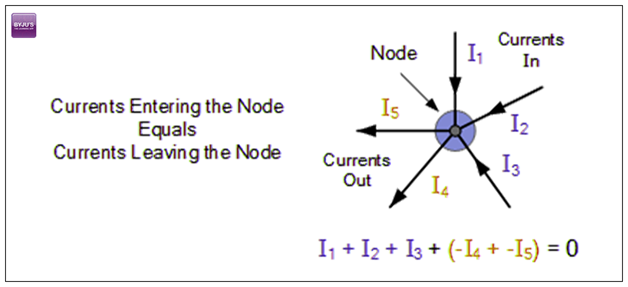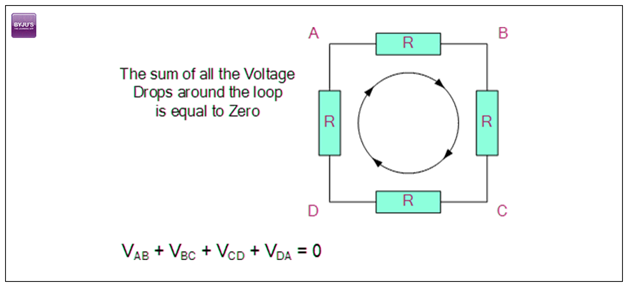# Kirchhoff's Law

## What is Kirchhoff’s Law?

Some relationships do exist between voltages and currents under various branches of an electrical circuit. These relationships are identified by the laws of which are known as Kirchhoff’s laws, derived by Guatov Robert  Kirchhoff. These laws help in calculating the electrical resistance of a complex network or impedance in case of AC and the current flow in different streams of the network.### What is Kirchhoff’s rule?

• According to the Junction rule in a circuit, the total of the currents in a junction is equal to the sum of currents outside the junction.
• According to the loop rule, the sum of the voltages around the closed loop is equal to null.

## Kirchhoff’s First Law

According to Kirchhoff’s Current Law,

The total current entering a junction or a node is equal to the charge leaving the node as no charge is lost.

Put differently, the algebraic sum of every current entering and leaving the node has to be null. This property of Kirchhoff law is commonly called as Conservation of charge wherein, I(exit) + I(enter) = 0.

### Kirchhoff’s Current LawIn the above figure, the currents I1, I2 and I3 entering the node are positive and two currents I4 and I5 are negative in values. This can be expressed in the form of an equation:

I1 + I2 + I3 – I4 – I5 = 0

The term Node refers to a junction or a connection of two or more current carrying routes like cables and other components. Kirchhoff’s current law can also be applied to analyze parallel circuits.

## Kirchhoff’s Second Law

According to Kirchhoff’s Voltage Law,

The voltage around a loop equals to the sum of every voltage drop in the same loop for any closed network and also equals to zero.

Put differently, the algebraic sum of every voltage in the loop has to be equal to zero and this property of Kirchhoff’s law is called as conservation of energy.

### Kirchhoff’s Voltage LawWhen you begin at any point of the loop and continue in the same direction, note the voltage drops in all the direction either negative or positive and return to the same point. It is essential to maintain the direction either counterclockwise or clockwise; else the final voltage value will not be equal to zero. The voltage law can also be applied in analyzing circuits in series.

When either AC circuits or DC circuits are analysed based on Kirchhoff’s circuit laws, you need to be clear with all the terminologies and definitions that describe the circuit components like: paths, nodes, meshes, and loops.